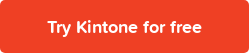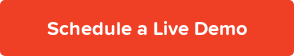January Kintone product updates will take effect on January 10, 2020.

New Features

IF Function Formula
IF Function is now available as a formula for Calculated and Text fields. The IF function gives two values, depending on the evaluation of the formula. If the evaluation results in “true, ” then it gives one value. If the evaluation is “false, ” it gives a different value.

Available operators: less than (<), less than or equal to (<=), greater than (>), greater than or equal to (>=), equal to (=), and not equal to (!=) or (<>).

Example: Shipping is equal to "\$5" if subtotal is "< \$20," "\$0" if subtotal is "≧ \$20."

Formula: IF(subtotal<20,5,0)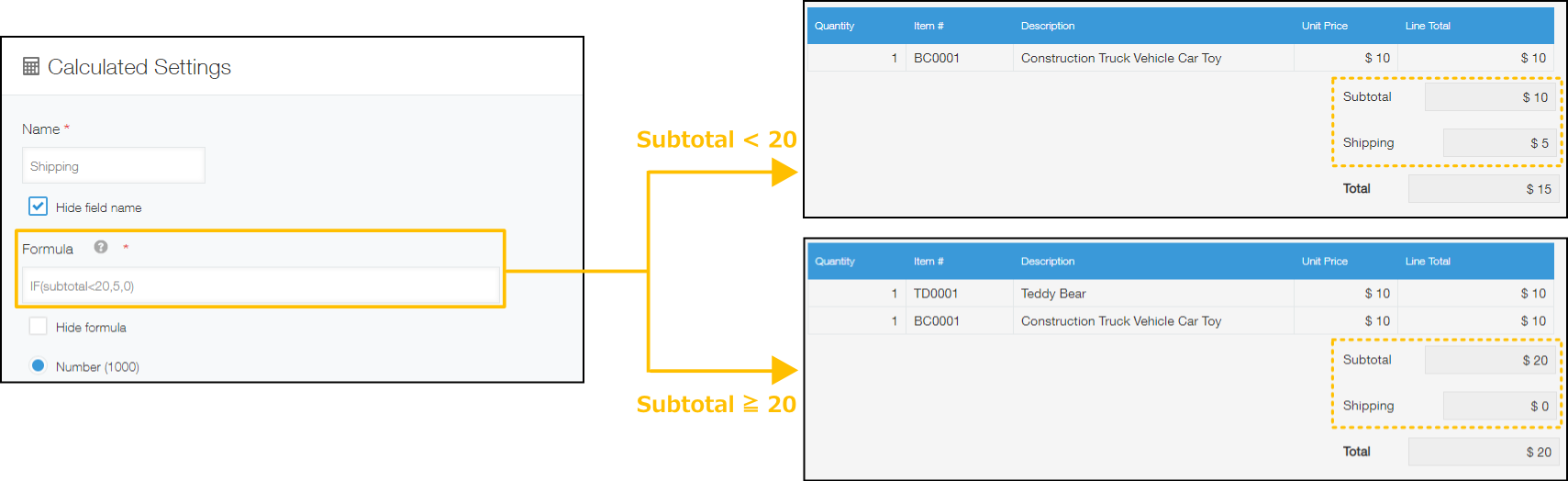Using IF with AND, OR, and NOT Functions:

AND Function:
IF(AND(logic1, logic2, logic3…), true value, false value)
If all of the logic is “true,” then it will return “true value.” If any of the logic is “false,” then it will return “false value.”

OR Function:
IF(OR(logic1, logic2, logic3…), true value, false value)
It will return “true value” if any of the logic is “true,” and “false value” if all of the logic is false.

NOT Function:
IF(NOT(logic), true value, false value)
When logical is “false,” it will return “true value.” When logical is “true,” it will return “false value.”

Example: If a student scores less than 80 on both Science and Mathematics exams, or even on one subject, the status should be “Fail.”

Formula: IF(AND(Science>=80, Mathematics>=80), "Pass","Fail")Using Drop-down and Radio button Fields in IF Formulas:
Example: Shipping should be \$10 if an order is international, free if domestic.

Formula: IF(country=”U.S.”,0,10)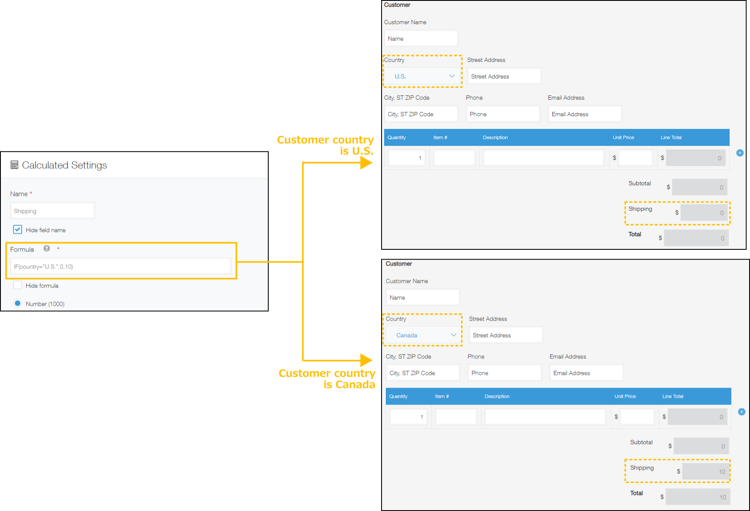Feature Improvements

Treating Blank Fields As Zeros In Formulas
Fields that are blank are evaluated as zero if a field is a number and as an empty string if a field is a text in a formula. There will no longer be "#N/A" errors for blank fields.

Before: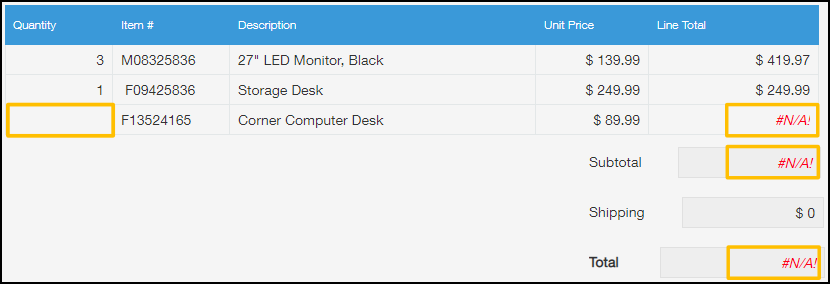After: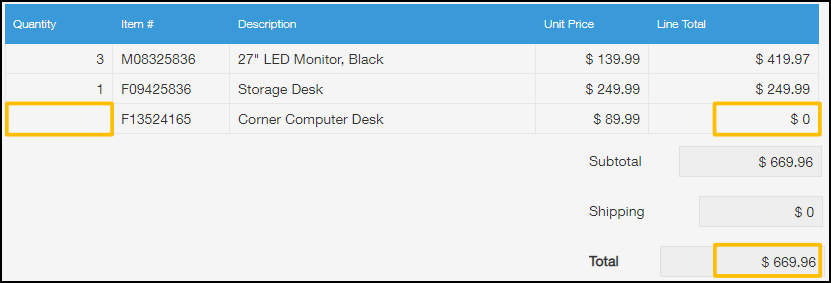Single Sign-On (SSO) For Kintone Mobile App
Added the ability to SSO into Kintone Mobile App with SAML authentication.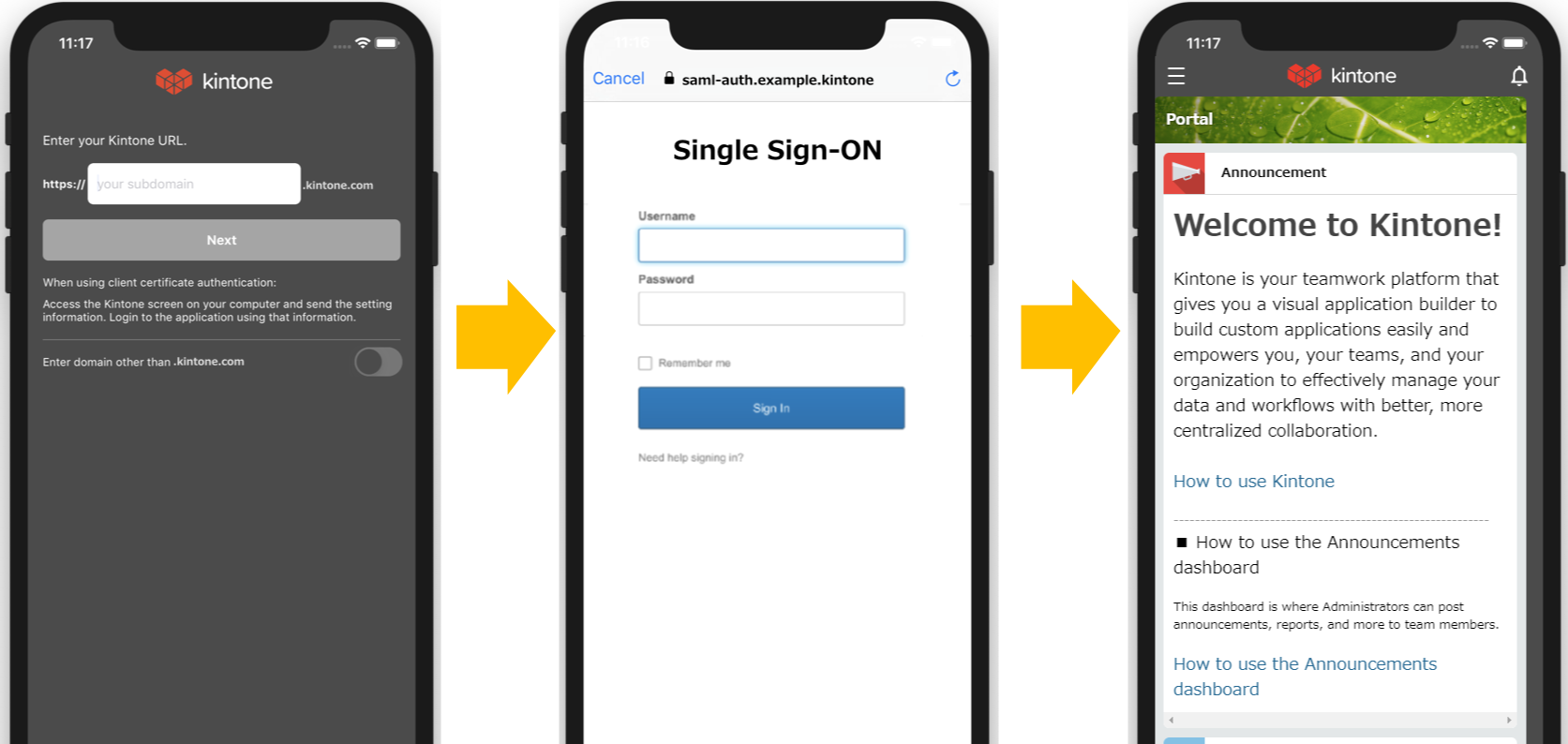Views Available On Mobile Will Be Displayed On The Top Of The List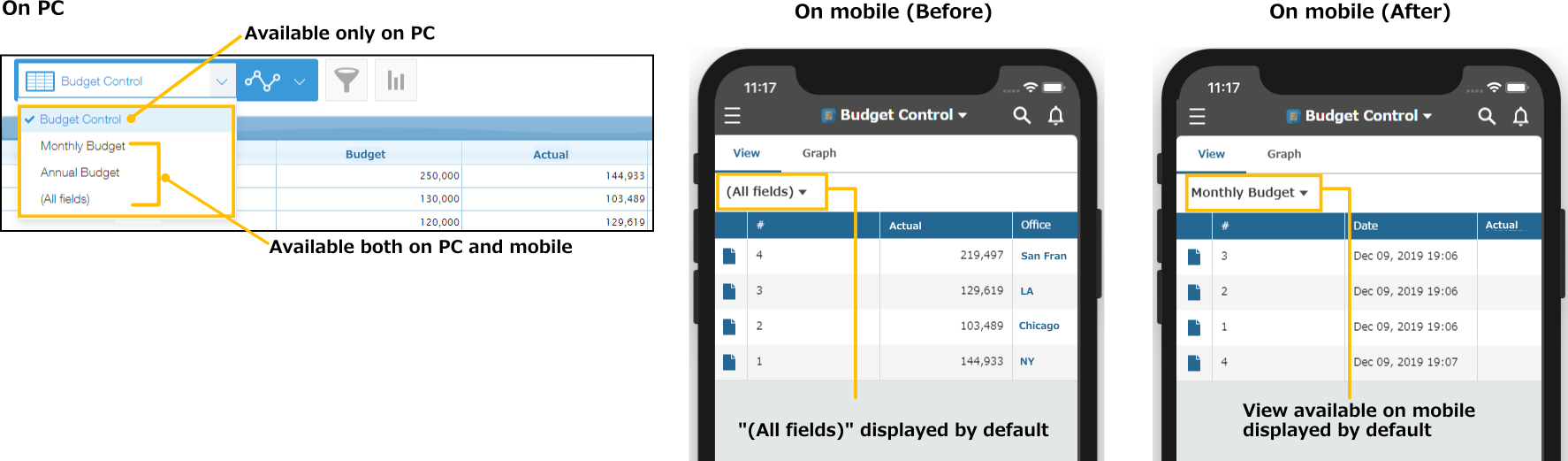### Subscribe to Blog